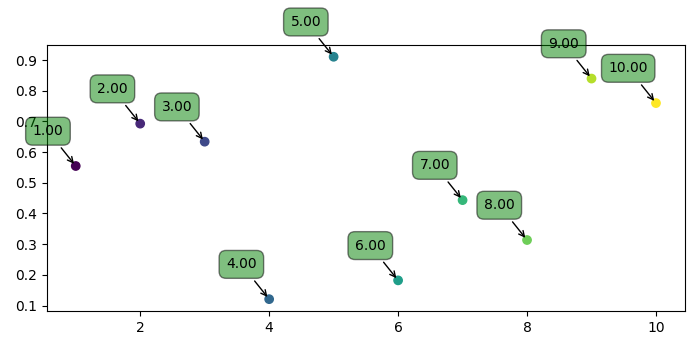# How to center an annotation horizontally over a point in Matplotlib?

MatplotlibServer Side ProgrammingProgramming

#### Python Data Science basics with Numpy, Pandas and Matplotlib

Most Popular

63 Lectures 6 hours

#### Data Visualization using MatPlotLib & Seaborn

11 Lectures 4 hours

#### MatPlotLib with Python

9 Lectures 2.5 hours

To center an annotation horizontally over a point, we can take the following steps−

• Create points for x and y using numpy.
• Create labels using xpoints.
• Use scatter() method to scatter the points.
• Iterate labels, xpoints and ypoints and annotate the plot with label, x and y with different properties, make horizontal alignment ha=center.
• To display the figure, use show() method.

## Example

import numpy as np
from matplotlib import pyplot as plt
plt.rcParams["figure.figsize"] = [7.00, 3.50]
plt.rcParams["figure.autolayout"] = True
xpoints = np.linspace(1, 10, 10)
ypoints = np.random.rand(10)
labels = ["%.2f" % i for i in xpoints]
plt.scatter(xpoints, ypoints, c=xpoints)
for label, x, y in zip(labels, xpoints, ypoints):
plt.annotate(
label,
xy=(x, y), xytext=(-20, 20),
textcoords='offset points', ha='center', va='bottom',
plt.show()